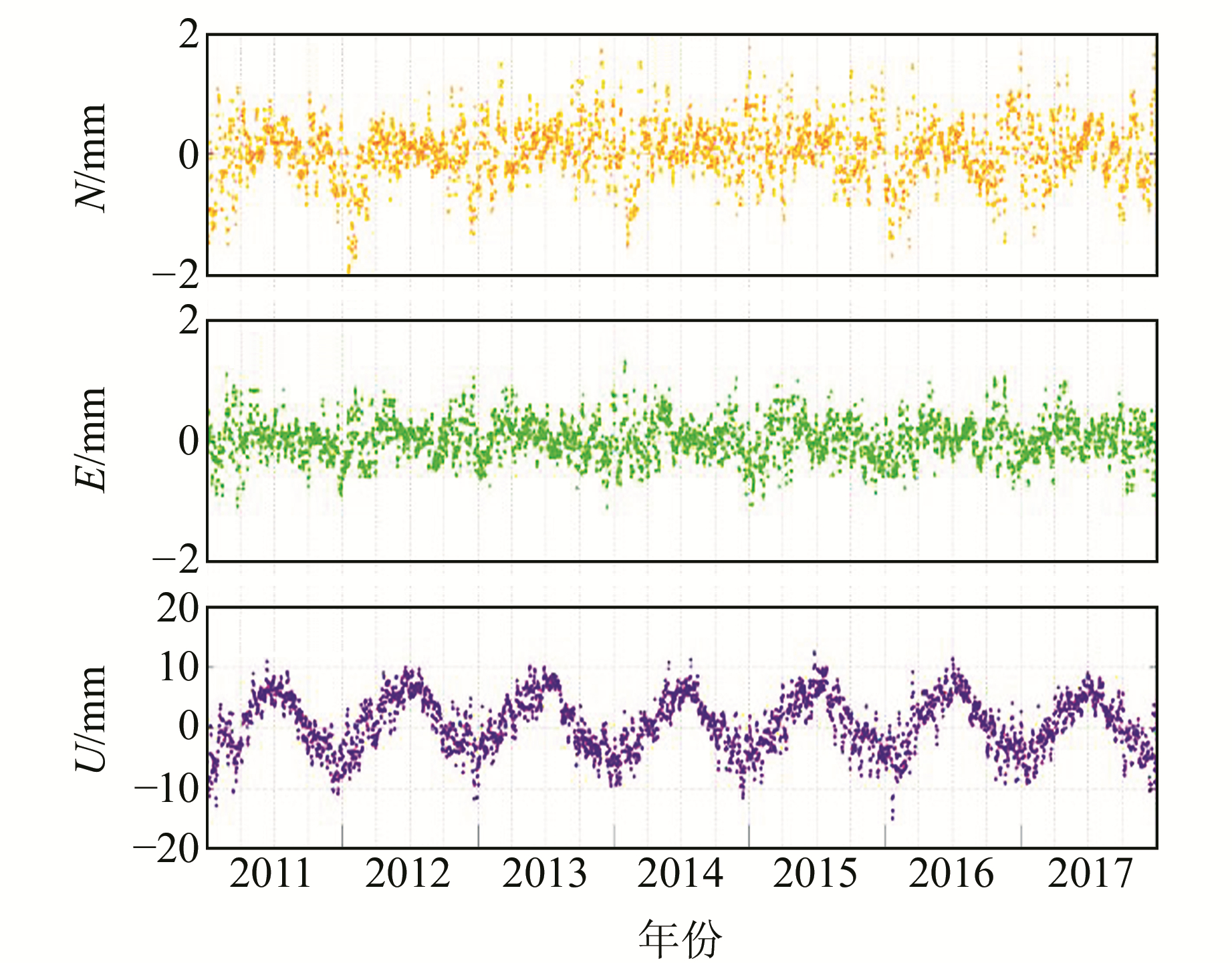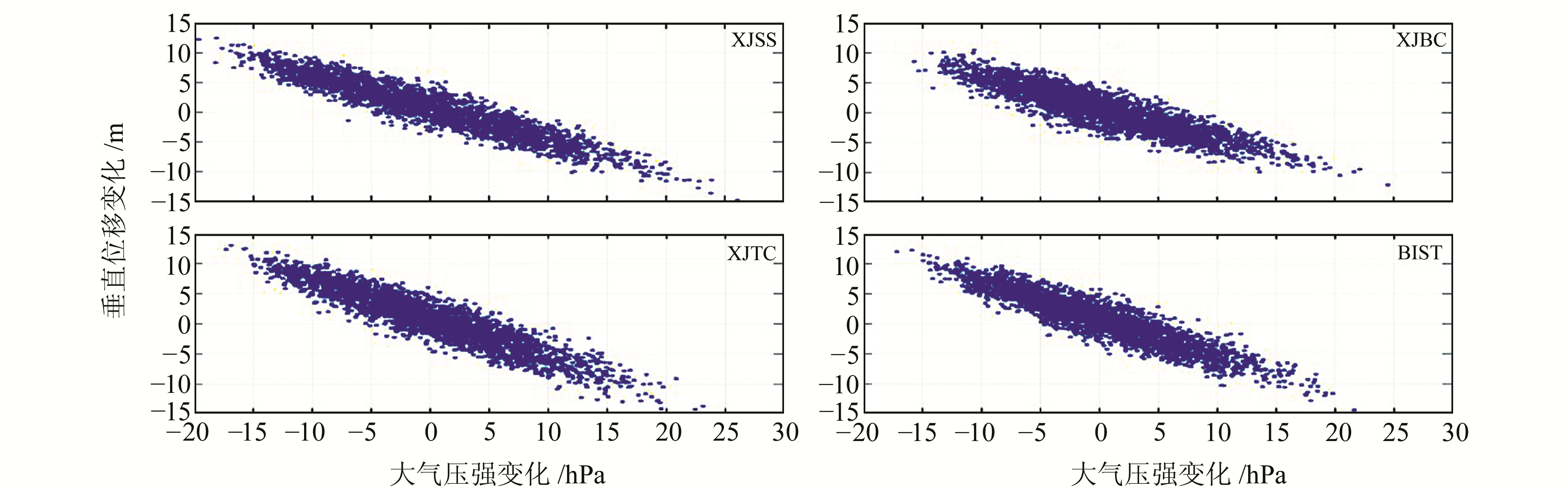﻿ 大气负荷变化对CORS位置影响分析与其移除研究文章快速检索 高级检索
 大地测量与地球动力学2019, Vol. 39Issue (11): 1159-1164  DOI: 10.14075/j.jgg.2019.11.012### 引用本文WANG Haitao, NIE Jianliang, TIAN Jie, et al. Influence Analysis of Atmospheric Load Change on Position of CORS and Its Removal Study[J]. Journal of Geodesy and Geodynamics, 2019, 39(11): 1159-1164.### Foundation support

National Natural Science Foundation of China, No.41574003, 41774004, 41474015, 41604001; Special Project of Basic Work of Science and Technology, Ministry of Science and Technology, No.2015FY210400; National Basic Surveying and Mapping Technological Project, No.2018KJ0205; Scientific Innovation Program of SHASG, No.2018-4.

### 第一作者简介

WANG Haitao, assistant engineer, majors in geodetic data processing, E-mail:1280181825@qq.com.

### 文章历史

1. 自然资源部大地测量数据处理中心，西安市友谊东路344号，710054;
2. 中国测绘科学研究院，北京市莲花池西路28号，100830;
3. 沈阳市勘察测绘研究院，沈阳市南三好街1号，110004

1 理论与方法

 $\begin{array}{l} \Delta q = {\rho _w}{h_w} = R{\rho _w}\sum\limits_{n = 1}^N {\sum\limits_{m = 0}^n {\left[ {\Delta C_{nm}^y\cos m\lambda + } \right.} } \\ \left. {\Delta S_{mn}^q\sin m\lambda } \right]{{\bar P}_{mn}}(\cos \theta ) \end{array}$ (1)

$k=3 \frac{\rho_{w}}{\rho_{e}}$，其规格化位系数的直接影响为$\Delta \bar{C}_{m n}=\frac{\kappa}{2 n+1} \Delta C_{m}^{q}, \Delta \bar{S}_{m n}=\frac{\kappa}{2 n+1} \Delta S_{m n}^{q}$, 则负荷位直接影响为：

 $\begin{array}{l} {\mathit{\Phi} ^d} = \kappa \frac{{GM}}{R}\sum\limits_{n = 2}^N {\frac{1}{{2n + 1}}} \sum\limits_{m = 0}^n {\left( {\Delta C_m^q\cos m\lambda + } \right.} \\ \left. {\Delta S_{mn}^q\sin m\lambda } \right){{\bar P}_{mn}}(\cos \theta ) \end{array}$ (2)

 $\begin{array}{l} {\mathit{\Phi} ^i} = \kappa \frac{{GM}}{R}\frac{1}{{2n + 1}}\sum\limits_{m = 0}^n {\left( {\Delta C_m^q\cos m\lambda + } \right.} \\ \left. {\Delta S_{mn}^q\sin m\lambda } \right){{\bar P}_{mn}}(\cos \theta ) \end{array}$ (3)

 ${\mathit{\Phi} ^r} = {\mathit{\Phi} ^d} + {\mathit{\Phi} ^i} = \sum\limits_{n = 2}^N {\left( {1 + k_n^\prime } \right)} {\mathit{\Phi} ^n}$ (4)

 $\begin{array}{l} e = \kappa \frac{{GM}}{{\gamma R\sin \theta }}\sum\limits_{n = 2}^N {\frac{{l_n^\prime }}{{2n + 1}}} \sum\limits_{m = 0}^n m \left( {\Delta C_{nm}^q\sin m\lambda - } \right.\\ \left. {\Delta S_{mn}^q\cos m\lambda } \right){{\bar P}_{mn}}(\cos \theta ) \end{array}$ (5)
 $\begin{array}{l} n = \kappa \frac{{GM}}{{\gamma R}}\sum\limits_{n = 2}^N {\frac{{l_n^\prime }}{{2n + 1}}} \sum\limits_{m = 0}^n {\left( {\Delta C_{nm}^q\cos m\lambda + } \right.} \\ \left. {\Delta S_{mn}^q\sin m\lambda } \right)\frac{\partial }{{\partial \theta }}{{\bar P}_{mn}}(\cos \theta ) \end{array}$ (6)

 $\begin{array}{l} r = \kappa \frac{{GM}}{{\gamma R}}\sum\limits_{n = 2}^N {\frac{{h_n^\prime }}{{2n + 1}}} \sum\limits_{m = 0}^n {\left( {\Delta C_m^q\cos m\lambda + } \right.} \\ \left. {\Delta S_{mn}^q\sin m\lambda } \right){{\bar P}_{mn}}(\cos \theta ) \end{array}$ (7)

2 数据处理与形变分析 2.1 数据来源及处理图 1 XJBE和XJBL测站时间序列 Fig. 1 Time series of XJBE and XJBL stations图 2 XJDS和XJFY测站滤去线性项后时间序列 Fig. 2 Time series of filtered XJDS and XJFY stations
2.2 大气负荷变化形变影响计算图 3 大气负荷形变对测站位置影响时间序列 Fig. 3 Time series of influence of atmospheric load deformation on stations
3 移去大气负荷影响分析

 ${X_{{\rm{RMS}}}} = \sqrt {\frac{{\sum\limits_{i = 1}^N {X_i^2} }}{N}} = \sqrt {\frac{{X_1^2 + X_2^2 + \cdots + X_{\rm{N}}^2}}{N}}$ (8)表 1 CORS位置时间序列RMS变化 Tab. 1 RMS change of CORS station location time series

 $U_{i}=K \cdot \Delta P_{i}$ (9)图 4 测站大气压强变化与垂向形变影响的关系 Fig. 4 Relationship between atmospheric pressure change and vertical deformation of station表 2 大气负荷变化对地壳垂直形变影响导纳值 Tab. 2 Admittance value of atmospheric load change on crustal vertical deformation
4 结语

  张诗玉, 钟敏. 地表流体变化对我国地壳垂直形变的影响[J]. 武汉大学学报:信息科学版, 2007, 32(5): 458-461 (Zhang Shiyu, Zhong Min. Vertical Crustal Displacements in China Due to Surface Fluid Changes[J]. Geomatics and Information Science of Wuhan University, 2007, 32(5): 458-461) (0)  李英冰.固体地球的环境变化响应[D].武汉: 武汉大学, 2003 (Li Yingbing. Solid Earth Response to Environmental Variation[D]. Wuhan : Wuhan University, 2003) http://cdmd.cnki.com.cn/Article/CDMD-10486-2006031873.htm (0)  张诗玉, 钟敏, 唐诗华, 等. 我国GPS基准站地壳垂直形变的大气负荷效应[J]. 武汉大学学报:信息科学版, 2006, 31(12): 1 090-1 093 (Zhang Shiyu, Zhong Min, Tang Shihua, et al. Vertical Crustal Displacements Due to Atmospheric Loading Effects at GPS Fiducial Stations in China[J]. Geomatics and Information Science of Wuhan University, 2006, 31(12): 1 090-1 093) (0)  罗少聪.大气负荷效应问题研究[D].武汉: 中国科学院测量与地球物理研究所, 2003 (Luo Shaocong. Study on the Loading Effects of the Atmospheric Pressure[D]. Wuhan: Institute of Geodesy and Geophysics, CAS, 2003) http://cdmd.cnki.com.cn/Article/CDMD-80057-2004041262.htm (0)  田云锋. 大气质量负荷对GPS基准站的影响[J]. 大地测量与地球动力学, 2010, 30(5): 64-67 (Tian Yunfeng. Effects of Atmospheric Mass Loading on GPS Fiducial Stations[J]. Journal of Geodesy and Geodynamics, 2010, 30(5): 64-67) (0)  王敏, 沈正康, 董大南. 非构造形变对GPS连续站位置时间序列的影响和修正[J]. 地球物理学报, 2005, 48(5): 1 045-1 052 (Wang Min, Shen Zhengkang, Dong Danan. Effects of Non-Tectonic Crustal Deformation on Continuous GPS Position Time Series and Correction to Them[J]. Chinese Journal of Geophysics, 2005, 48(5): 1 045-1 052) (0)  王伟, 党亚民, 章传银, 等. 基于CORS站网监测三峡地区陆地水负荷对地壳形变和重力变化的影响[J]. 地球物理学报, 2017, 60(3): 962-971 (Wang Wei, Dang Yamin, Zhang Chuanyin, et al. Monitoring of Crustal Deformation and Gravity Variation from Terrestrial Water Loading in the Three Gorges Region by the CORS Network[J]. Chinese Journal of Geophysics, 2017, 60(3): 962-971) (0)  党亚民, 章传银, 陈俊勇, 等. 现代大地测量基准[M]. 北京: 测绘出版社, 2015 (Dang Yamin, Zhang Chuanyin, Chen Junyong, et al. Modern Geodetic Datum[M]. Beijing: Surveying and Mapping Press, 2015) (0)  章传银, 王伟, 甘卫军, 等. 利用CORS站网监测三峡地区环境负荷引起的地壳形变与重力场时空变化[J]. 武汉大学学报:信息科学版, 2018, 43(9): 1 287-1 294 (Zhang Chuanyin, Wang Wei, Gan Weijun, et al. Monitoring Temporal and Spatial Changes of Crustal Deformation and Gravity Field Caused by Environmental Load in the Three Gorges Reservoir Region Based on CORS Network[J]. Geomatics and Information Science of Wuhan University, 2018, 43(9): 1 287-1 294) (0)  Longman I M. A Green's Function for Determining the Deformation of the Earth under Surface Mass Loads[J]. Journal of Geophysical Research, 1962, 68(2): 485-496 (0)  Farrell W. Deformation of the Earth by Surface Loads[J]. Reviews of Geophysics and Space Physics, 1972, 10(3): 761-797 DOI:10.1029/RG010i003p00761 (0)  Spratt R S. Modeling the Effect of Atmospheric Pressure Variations on Gravity[J]. Geophysical Journal of the Royal Astronomical Society, 2010, 71(1): 173-186 (0)  沈迎春, 闫昊明, 彭鹏, 等. 质量负荷引起地表形变的格林函数和球谐函数方法对比研究[J]. 武汉大学学报:信息科学版, 2017, 42(7): 1 008-1 014 (Shen Yingchun, Yan Haoming, Peng Peng, et al. Comparative Study of Green's Function and Spherical Harmonic Function Methods on Surface Deformation Caused by Mass Loading[J]. Geomatics and Information Science of Wuhan University, 2017, 42(7): 1 008-1 014) (0)  周旭华, 吴斌. 大气、陆地水储量和海水质量分布变化与地球低阶引力场球谐系数的关系[J]. 天文学报, 2002, 43(3): 327-332 (Zhou Xuhua, Wu Bin. Changes of Atmosphere, Continental Water and Oceanic Mass Distribution in Relation with Low Degree Harmonic Coefficients in the Earth's Gravitational Field[J]. Acta Astronomica, 2002, 43(3): 327-332 DOI:10.3321/j.issn:0001-5245.2002.03.011) (0)  Ray R D, Ponte R M. Barometric Tides from ECMWF Operational Analyses[J]. Annales Geophysicae, 2003, 21(8): 1 897-1 910 DOI:10.5194/angeo-21-1897-2003 (0)  Vandam T M, Blewitt G, Heflin M B. Atmospheric Pressure Loading Effects on Global Positioning System Coordinate Determinations[J]. Journal of Geophysical Research: Solid Earth, 1994, 99(B12): 23 939-23 950 DOI:10.1029/94JB02122 (0)
Influence Analysis of Atmospheric Load Change on Position of CORS and Its Removal Study
WANG Haitao1NIE Jianliang1     TIAN Jie1     WANG Wei2     GUO Xinwei1     JIN Xinyang1     TAI He3     CHENG Xiawei1
1. Geodetic Data Processing Centre, MNR, 334 East-Youyi Road, Xi'an 710054, China;
2. Chinese Academy of Surveying and Mapping, 28 West-Lianhuachi Road, Beijing 100830, China;
3. Shenyang Research Institute of Surveying and Mapping, 1 South-Sanhao Street, Shenyang 110004, China
Abstract: Based on the global atmospheric model and the time series data of CORS location, we calculate the influence of atmospheric load on the crustal deformation at CORS locations using the spherical harmonic coefficient method. The results show that the influence of atmospheric load on the vertical direction of CORS location in the study area is -15.9 to 13.2 mm, and the influence on horizontal deformation is 0 to 2.7 mm. According to the change of RMS value before and after removing the influence of atmospheric load on CORS, it is concluded that removing the influence of atmospheric load on the horizontal direction of CORS time series has little improvement, but has obvious improvement on the vertical direction. According to the least square method, the crustal vertical deformation admittance(regression coefficient) of the study area varies from -0.75 to -0.43 mm/hPa. The research methods and results of this paper can be used for reference in the separation and correction of atmospheric load in CORS.
Key words: atmospheric load; CORS; spherical harmonic coefficient method; vertical deformatio